# High School Algebra: Quadratic Equations Chapter Exam

Exam Instructions:

Choose your answers to the questions and click 'Next' to see the next set of questions. You can skip questions if you would like and come back to them later with the yellow "Go To First Skipped Question" button. When you have completed the practice exam, a green submit button will appear. Click it to see your results. Good luck!

### Page 1

#### Question 1 1. What is the b-value of the quadratic equation below?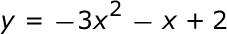#### Question 4 4. Find the roots of the equation.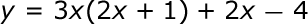### Page 2

#### Question 6 6. Use the quadratic formula to find the solutions to the equation below.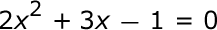#### Question 7 7. Which statement is true about the inequality below?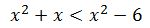#### Question 8 8. Rewrite the quadratic below in standard form: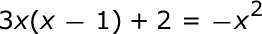### Page 4

#### Question 17 17. Which of the following are the solutions to the equation below?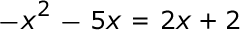#### Question 20 20. Solve the following equation.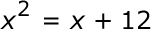### Page 5

#### Question 21 21. Solve this inequality.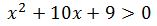#### Question 22 22. Solve the following inequality.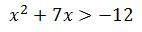#### Question 23 23. What are the zeros in the following quadratic equation?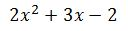#### Question 25 25. Find the roots of the equation below.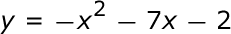#### High School Algebra: Quadratic Equations Chapter Exam Instructions

Choose your answers to the questions and click 'Next' to see the next set of questions. You can skip questions if you would like and come back to them later with the yellow "Go To First Skipped Question" button. When you have completed the practice exam, a green submit button will appear. Click it to see your results. Good luck!

Support